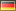The commands A1.<x>=AffineSpace(1, QQ) X=A1.subscheme([x^1789+x]) Q=X() Q.multiplicity() result in 1789. There seems to be a bug in the multiplicity command for subschemes of the line.2 retaggedrburing7733 ●4 ●57 ●159 http://rburing.nl/
The commands A1.<x>=AffineSpace(1, QQ) X=A1.subscheme([x^1789+x]) Q=X() Q.multiplicity() result in 1789. There seems to be a bug in the multiplicity command for subschemes of the line.